SBOS784C November   2016  – January 2019

PRODUCTION DATA.

1. Features
2. Applications
3. Description
4. Revision History
5. Device Comparison Table
6. Pin Configuration and Functions
7. Specifications
8. Detailed Description
1. 8.1 Overview
2. 8.2 Functional Block Diagram
3. 8.3 Feature Description
4. 8.4 Device Functional Modes
9. Application and Implementation
1. 9.1 Application Information
2. 9.2 Typical Application
10. 10Power Supply Recommendations
11. 11Layout
12. 12Device and Documentation Support
1. 12.1 Device Support
1. 12.1.1 Third-Party Products Disclaimer
2. 12.1.2 Development Support
2. 12.2 Documentation Support
5. 12.5 Community Resources
7. 12.7 Electrostatic Discharge Caution
8. 12.8 Glossary
13. 13Mechanical, Packaging, and Orderable Information

• D|8
• DCK|5
• DBV|5
• D|8
• DCK|5

### 7.8 Typical Characteristics

at VS = ±18 V, VCM = VS / 2, RLOAD = 10 kΩ connected to VS / 2, and CL = 100 pF (unless otherwise noted)

### Table 1. Characteristic Performance Measurements

DESCRIPTION FIGURE
Offset Voltage Production Distribution Figure 1
Offset Voltage vs Common-Mode Voltage Figure 2
Offset Voltage vs Common-Mode Voltage (Upper Stage) Figure 3
Input Bias Current vs Temperature Figure 4
Output Voltage Swing vs Output Current (Maximum Supply) Figure 5
CMRR and PSRR vs Frequency (Referred-to-Input) Figure 6
0.1-Hz to 10-Hz Noise Figure 7
Input Voltage Noise Spectral Density vs Frequency Figure 8
Quiescent Current vs Supply Voltage Figure 9
Open-Loop Gain and Phase vs Frequency Figure 10
Closed-Loop Gain vs Frequency Figure 11
Open-Loop Output Impedance vs Frequency Figure 12
Small-Signal Overshoot vs Capacitive Load Figure 13, Figure 14
No Phase Reversal Figure 15
Small-Signal Step Response (10 mV) Figure 16, Figure 17
Large-Signal Step Response Figure 18, Figure 19
Large-Signal Settling Time Figure 20, Figure 21
Short-Circuit Current vs Temperature Figure 22
Maximum Output Voltage vs Frequency Figure 23
EMIRR IN+ vs Frequency Figure 24
at VS = ±18 V, VCM = VS / 2, RLOAD = 10 kΩ connected to VS / 2, and CL = 100 pF (unless otherwise noted)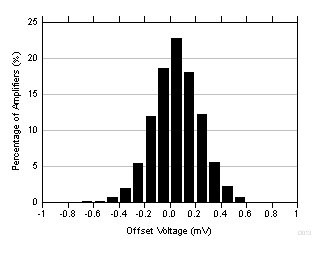Distribution taken from 5185 amplifiers
Figure 1. Offset Voltage Production Distribution Histogram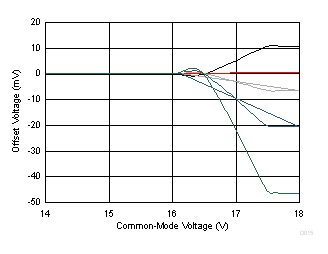5 typical units shown, VS = ±18 V
Figure 3. Offset Voltage vs Common-Mode Voltage
(Upper Stage)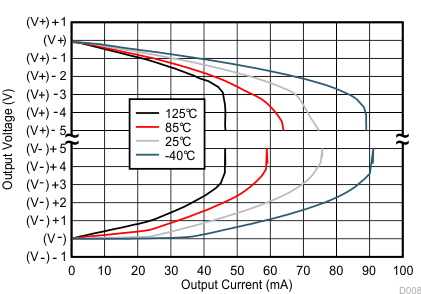Figure 5. Output Voltage Swing vs Output Current (Maximum Supply)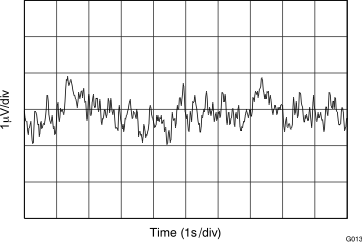Figure 7. 0.1-Hz to 10-Hz Noise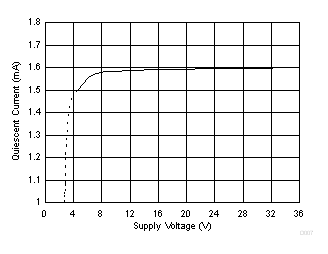Figure 9. Quiescent Current vs Supply Voltage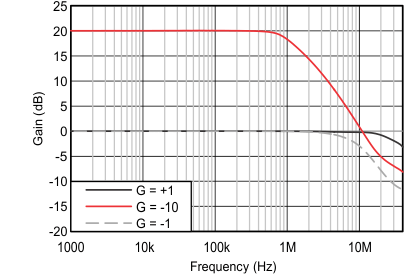Figure 11. Closed-Loop Gain vs Frequency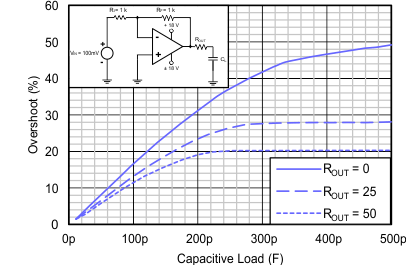100-mV output step, G = –1
Figure 13. Small-Signal Overshoot vs Capacitive Load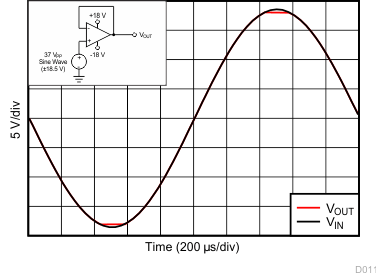Figure 15. No Phase Reversal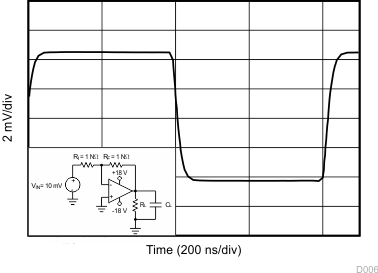RL = 1 kΩ CL = 10 pF 10-mV step
Figure 17. Small-Signal Step Response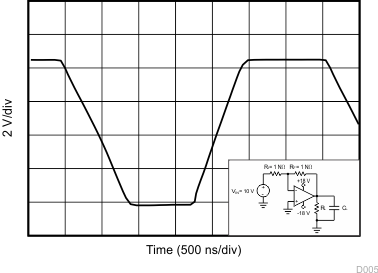RL = 1 kΩ CL = 10 pF
Figure 19. Large-Signal Step Response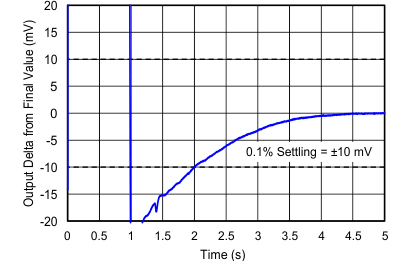10-V negative step G = 1 CL = 10 pF
Figure 21. Large-Signal Settling Time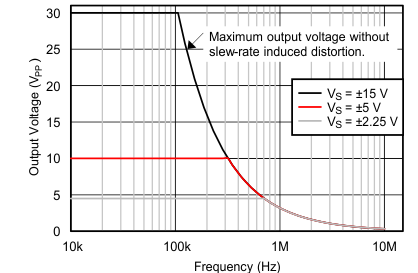Figure 23. Maximum Output Voltage vs Frequency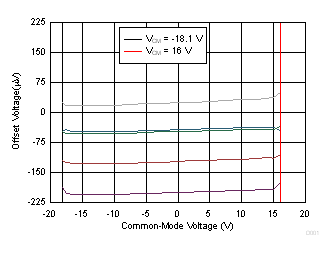5 typical units shown, VS = ±18 V
Figure 2. Offset Voltage vs Common-Mode Voltage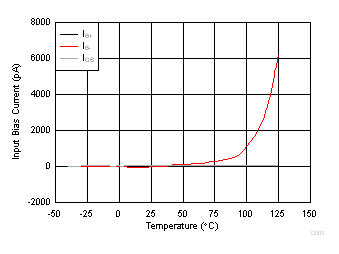Figure 4. Input Bias Current vs TemperatureFigure 6. CMRR and PSRR vs Frequency (Referred-to-Input)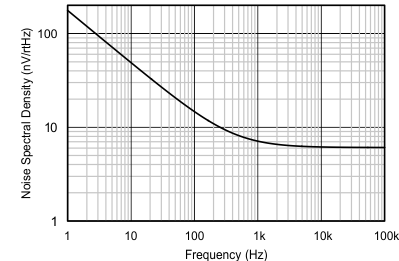Figure 8. Input Voltage Noise Spectral Density vs Frequency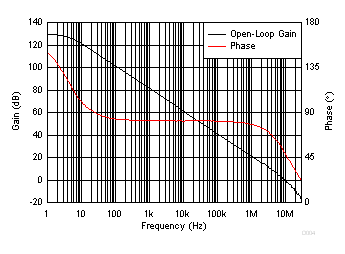Figure 10. Open-Loop Gain and Phase vs Frequency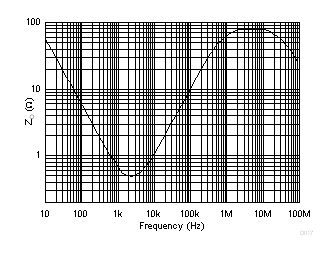Figure 12. Open-Loop Output Impedance vs Frequency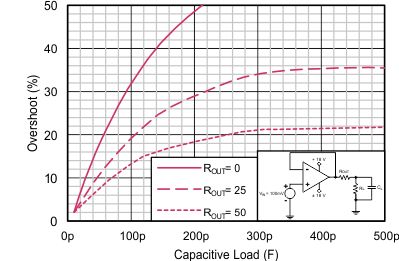100-mV output step, G = 1
Figure 14. Small-Signal Overshoot vs Capacitive Load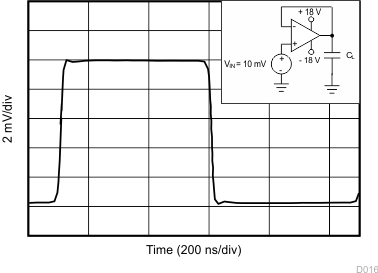CL = 10 pF 10-mV step
Figure 16. Small-Signal Step Response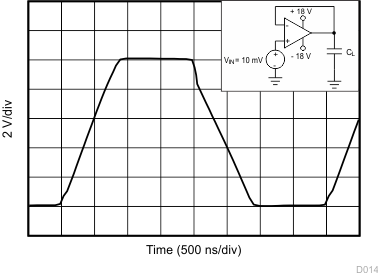CL = 10 pF
Figure 18. Large-Signal Step Response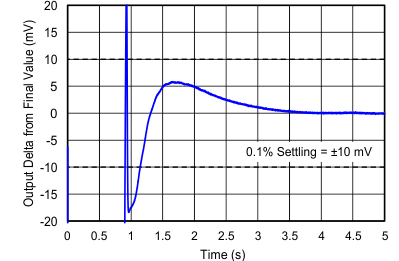10-V positive step G = 1 CL = 10 pF
Figure 20. Large-Signal Settling Time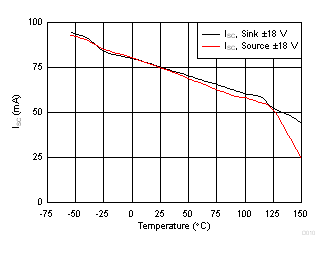Figure 22. Short-Circuit Current vs Temperature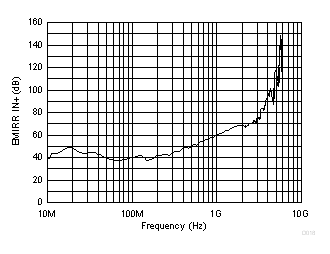PRF = –10 dBm VSUPPLY = ±18 V VCM = 0 V
Figure 24. EMIRR IN+ vs Frequency